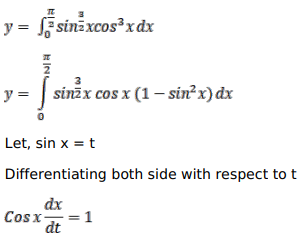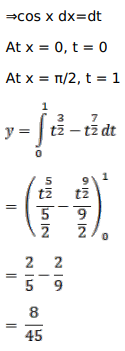# Mark against the correct answer in the following:

Question:

Mark $(\sqrt{ })$ against the correct answer in the following:

$\int_{0}^{\pi / 2}(\sqrt{\sin x} \cos x)^{3} d x=?$

A. $\frac{2}{9}$

B. $\frac{2}{15}$

C. $\frac{8}{45}$

D. $\frac{5}{2}$

Solution: